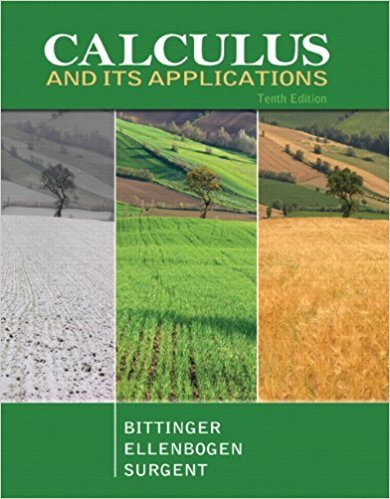×
×

# Solutions for Chapter 2.7: Implicit Differentiation and Related Rates## Full solutions for Calculus and Its Applications | 10th Edition

ISBN: 9780321694331Solutions for Chapter 2.7: Implicit Differentiation and Related Rates

Solutions for Chapter 2.7
4 5 0 254 Reviews
25
3
##### ISBN: 9780321694331

Since 64 problems in chapter 2.7: Implicit Differentiation and Related Rates have been answered, more than 24241 students have viewed full step-by-step solutions from this chapter. Calculus and Its Applications was written by and is associated to the ISBN: 9780321694331. This expansive textbook survival guide covers the following chapters and their solutions. This textbook survival guide was created for the textbook: Calculus and Its Applications, edition: 10. Chapter 2.7: Implicit Differentiation and Related Rates includes 64 full step-by-step solutions.

Key Calculus Terms and definitions covered in this textbook
• Blocking

A feature of some experimental designs that controls for potential differences between subject groups by applying treatments randomly within homogeneous blocks of subjects

• Combinatorics

A branch of mathematics related to determining the number of elements of a set or the number of ways objects can be arranged or combined

• De Moivre’s theorem

(r(cos ? + i sin ?))n = r n (cos n? + i sin n?)

• Factored form

The left side of u(v + w) = uv + uw.

• First-degree equation in x , y, and z

An equation that can be written in the form.

• General form (of a line)

Ax + By + C = 0, where A and B are not both zero.

• Inferential statistics

Using the science of statistics to make inferences about the parameters in a population from a sample.

• Inverse tangent function

The function y = tan-1 x

• kth term of a sequence

The kth expression in the sequence

• Lower bound for real zeros

A number c is a lower bound for the set of real zeros of ƒ if ƒ(x) Z 0 whenever x < c

• Multiplicity

The multiplicity of a zero c of a polynomial ƒ(x) of degree n > 0 is the number of times the factor (x - c) (x - z 2) Á (x - z n)

• Natural logarithmic function

The inverse of the exponential function y = ex, denoted by y = ln x.

• Quartile

The first quartile is the median of the lower half of a set of data, the second quartile is the median, and the third quartile is the median of the upper half of the data.

• Quotient of functions

a ƒ g b(x) = ƒ(x) g(x) , g(x) ? 0

• Real number

Any number that can be written as a decimal.

• Root of an equation

A solution.

• Speed

The magnitude of the velocity vector, given by distance/time.

• Window dimensions

The restrictions on x and y that specify a viewing window. See Viewing window.

• y-coordinate

The directed distance from the x-axis xz-plane to a point in a plane (space), or the second number in an ordered pair (triple), pp. 12, 629.

• yz-plane

The points (0, y, z) in Cartesian space.

×